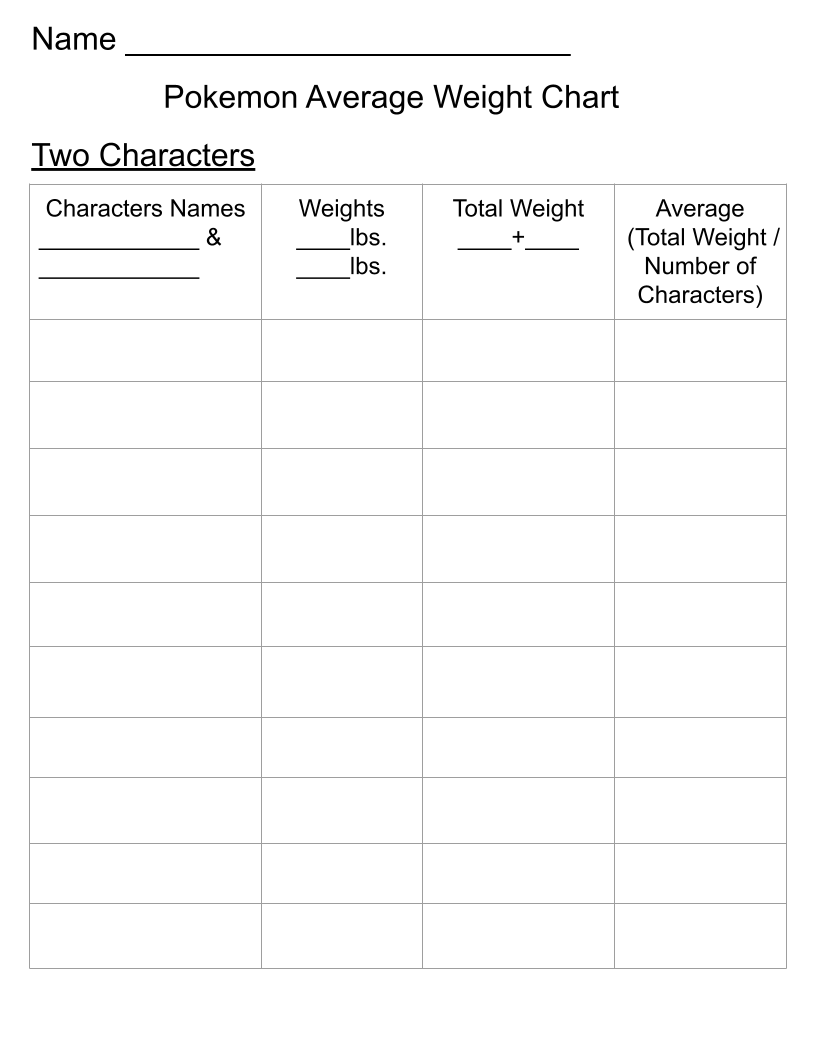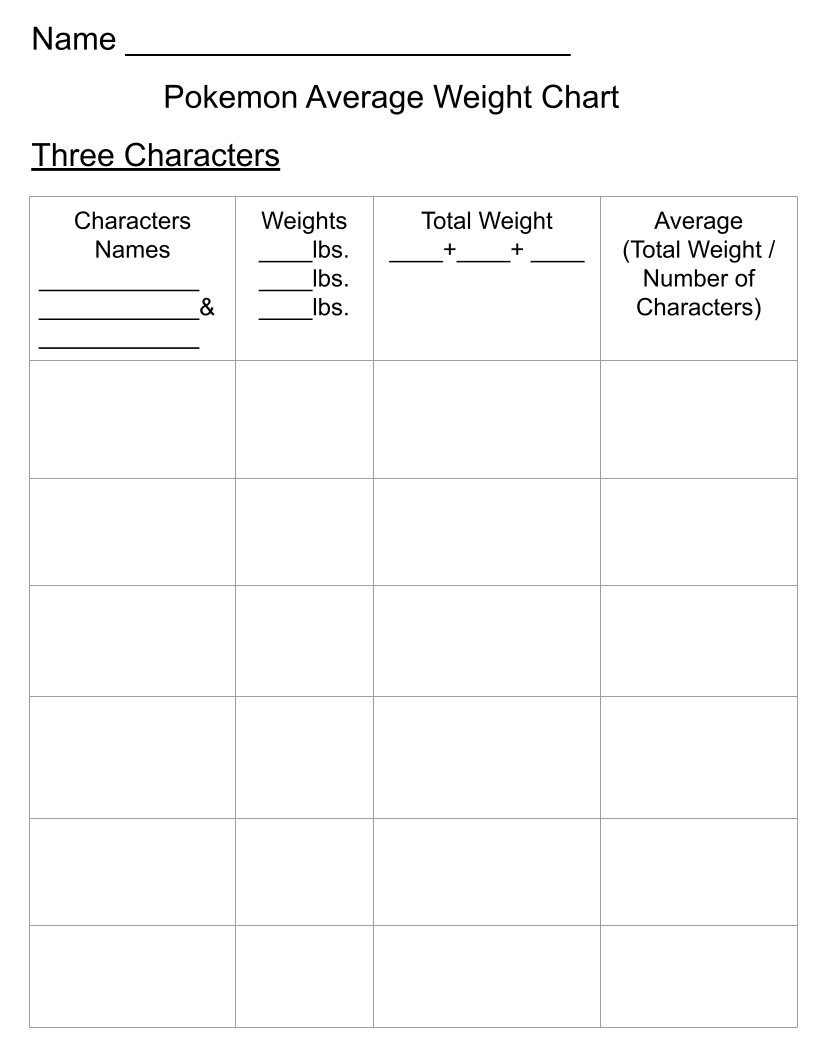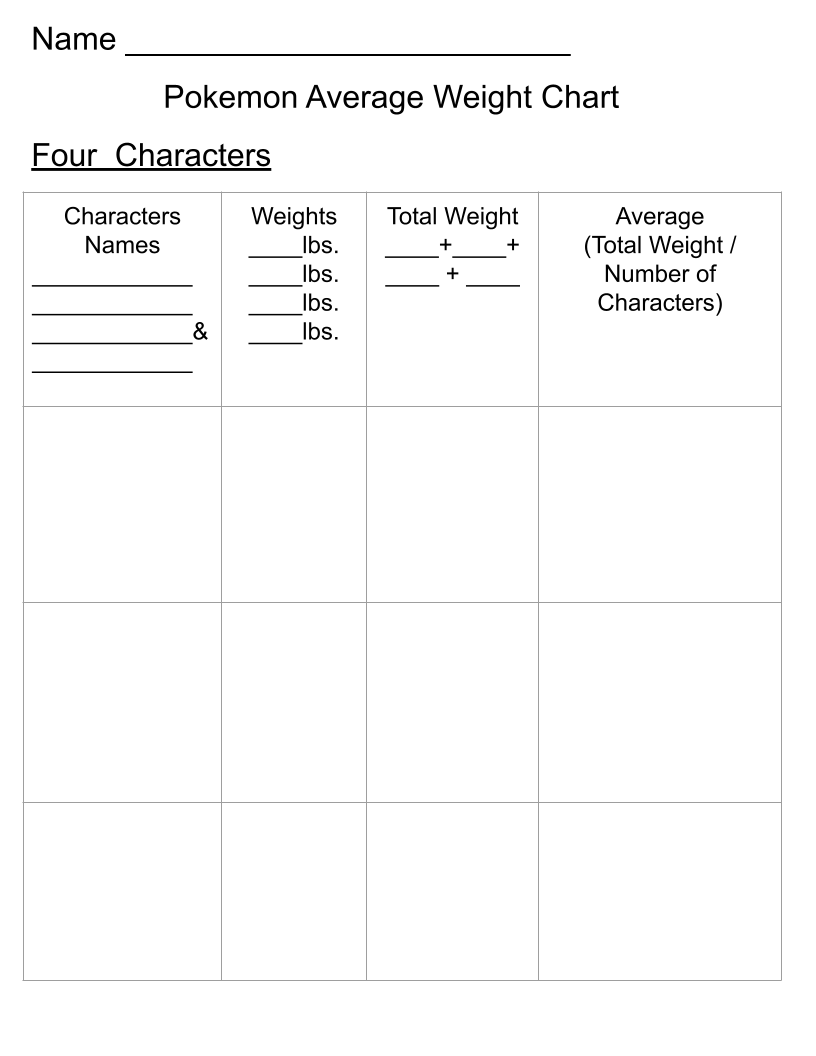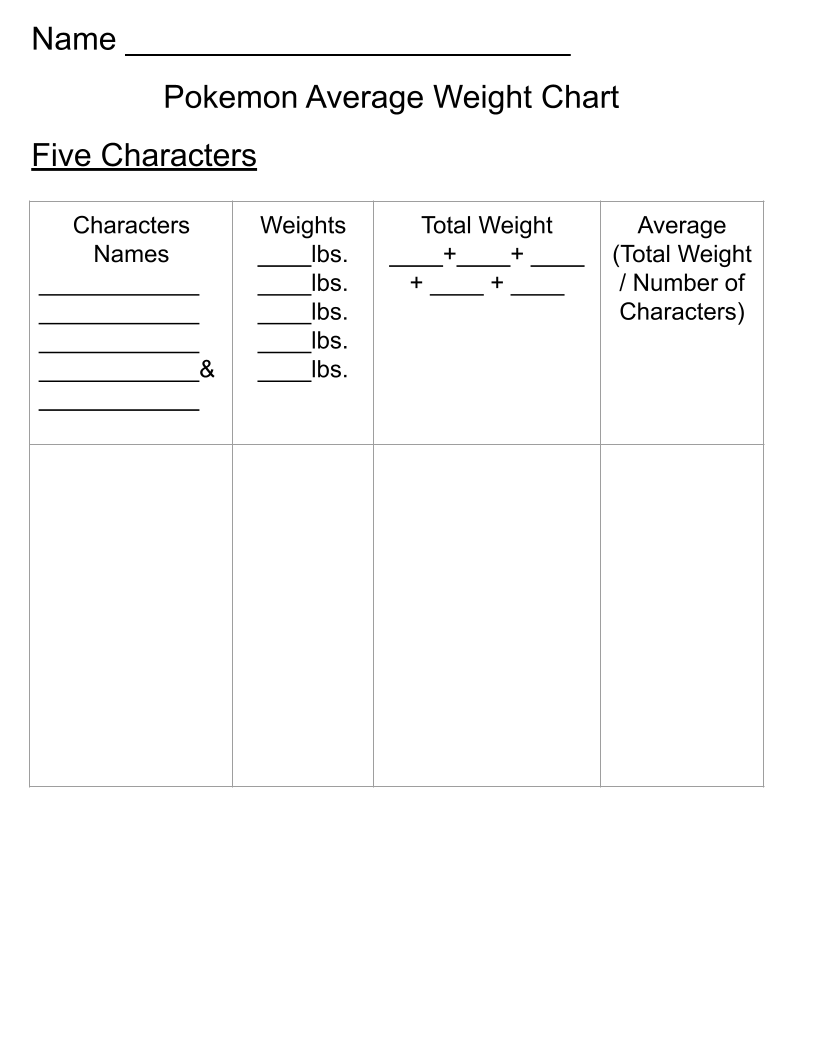# Finding Averages Pokemon Trading Card Lesson Plan

Subject: Math

Note:

• Students must know how to add decimals before completing this lesson.

Objective:

Students will be able to find the average weight of Pokemon characters.

Anticipatory Set:

• Ask students to think of what it means to find the average of something.
• Have partners discuss what average means.
• Share out and write responses on the board.

Instruction:

• Explain that students will be learning how to find averages of sets of data today.
• Begin with asking students to count the number of letters in their first name.
• Record the numbers on the board.
• Model how to add the numbers and then divide by the total number of students in the class.
• Explain those are the steps for finding the average of a set of data.
• Show two Pokemon cards and write the weights of the characters on the board.
• Model how to add the two weights together and then divide by the total number of data (2) to find the average weight of those two characters.
• Model the same process with three cards.
• Demonstrate how to fill this information into the Pokemon Average Weight Chart.

Guided Practice:

• Give students a list of numbers (with decimals to the tenths column) to find the averages using mini-whiteboards or pencil & paper.
• Have the students turn their whiteboards/paper to show you their answers.
• Continue practicing finding averages with random numbers.

Independent Practice:

• Pass out five Pokemon cards to each student.
• Have the students fill in the chart by picking two characters to find their average weight.
• Continue filling in that section by choosing two different characters to find their average weight.
• Continue until all possible combinations of two characters have been completed.
• Have students fill in the chart by picking three characters to find their average weights.
• Continue filling in that section by choosing three different characters to find their average weight.
• Continue until all possible combinations of three characters have been completed.
• Have students finish filling in the chart by finding the average weights with all the combinations of four characters and all the combinations of five characters.

Closure:

• Exit ticket:  Give students three or four new Pokemon cards to find the average of the characters’ weights on a half sheet of paper.